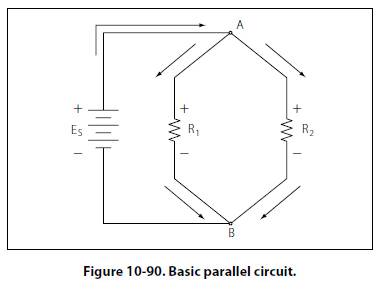Parallel DC Circuits Overview A circuit in which two of more electrical resistances or loads are connected across the same voltage source is called a parallel circuit. The primary difference between the series circuit and the parallel circuit is that more than one path is provided for the current in the parallel circuit. Each of these parallel paths is called a branch. The minimum requirements for a parallel circuit are the following: A power source. Conductors. A resistance or load for each current path. Two or more paths for current flow. Figure 10-90 depicts the most basic parallel circuit. Current flowing out of the source divides at point A in the diagram and goes through R1 and R2. As more branches are added to the circuit, more paths for the source current are provided.Voltage Drops The first point to understand is that the voltage across any branch is equal to the voltage across all of the other branches. Total Parallel Resistance The voltage across any branch is equal to the voltage across all of the other branches. The parallel circuit consists of two or more resistors connected in such a way as to allow current flow to pass through all of the resistors at once. This eliminates the need for current to pass one resistor before passing through the next. When resistors are connected in parallel, the total resistance of the circuit decreases. The total resistance of a parallel combination is always less than the value of the smallest resistor in the circuit. In the series circuit, the current has to pass through the resistors one at a time. This gave a resistance to the current equal the sum of all the resistors. In the parallel circuit, the current has several resistors that it can pass through, actually reducing the total resistance of the circuit in relation to any one resistor value. The amount of current passing through each resistor will vary according to its individual resistance. The total current of the circuit will then be the sum of the current in all branches. It can be determined by inspection that the total current will be greater than that of any given branch. Using Ohm’s law to calculate the resistance based on the applied voltage and the total current, it can be determined that the total resistance is less than any individual branch. An example of this is if there was a circuit with a 100 O resistor and a 5 O resistor; while the exact value must be calculated, it still can be said that the combined resistance between the two will be less than the 5 O.
 ©AvStop Online Magazine                                                                                                                                                      Contact Us              Return To Books